Courses

# Test: Design For Static And Dynamic Load (Level - 1)

## 10 Questions MCQ Test Machine Design | Test: Design For Static And Dynamic Load (Level - 1)

Description
This mock test of Test: Design For Static And Dynamic Load (Level - 1) for Mechanical Engineering helps you for every Mechanical Engineering entrance exam. This contains 10 Multiple Choice Questions for Mechanical Engineering Test: Design For Static And Dynamic Load (Level - 1) (mcq) to study with solutions a complete question bank. The solved questions answers in this Test: Design For Static And Dynamic Load (Level - 1) quiz give you a good mix of easy questions and tough questions. Mechanical Engineering students definitely take this Test: Design For Static And Dynamic Load (Level - 1) exercise for a better result in the exam. You can find other Test: Design For Static And Dynamic Load (Level - 1) extra questions, long questions & short questions for Mechanical Engineering on EduRev as well by searching above.
QUESTION: 1

### Which strength of the material is to be considered for design of a ductile component under cyclic load?

Solution: Endurance strength is to be considered for design of a component under cyclic load. The fatigue or endurance limit of a material is defined as the maximum amplitude of completely reversed stress that the standard specimen can sustain for an unlimited number of cycles without fatigue failure.

QUESTION: 2

### The relationship between notch sensitivity factor (q), theoretical stress concentration factor(kt) and fatigue (or) form stress concentration (kf) is given

Solution: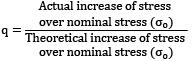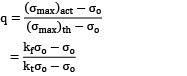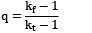Note:

Actual stress concentration factor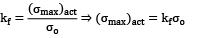Theoretical stress concentration factor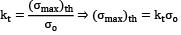QUESTION: 3

### Endurance limit is determined by a test, applying

Solution:
QUESTION: 4

In a semi-infinite flat plate shown in the figure, the theoretical stress concentration factor kt for an elliptical hole of major axis 2a and minor axis 2b is given by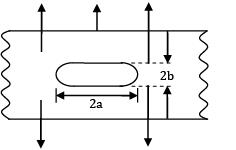Solution:
QUESTION: 5

Fatigue of a component is due to

Solution: Fatigue of a component is due to cyclic load. Fatigue occurs when a material is subjected to repeated loading and unloading.

QUESTION: 6

Endurance limit of a component

Solution:
QUESTION: 7

Number of cycles of repetitions for low cycle fatigue in steel is

Solution:
QUESTION: 8

To design a component of ductile material under variable loading, stress concentration factor is applied to

Solution:
QUESTION: 9

The maximum value of stress concentration factor in an infinite plate with a circular hole under uniaxial tension is

Solution: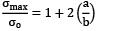For circular, a = b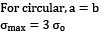QUESTION: 10

Which of the following is not the correct procedure to increase the fatigue limit

Solution: Fatigue limit of material can be increased by
• By applying residual compressive stress (under stress)

• By surface finishing

• By shot peening

• Cold working